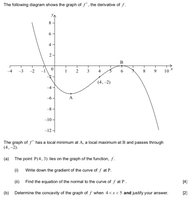# Finding the gradient and concavity.

#### Eumwilkinson

##### New member
Hicompletely lost on this question. I don’t understand how to find the gradient ai), even though it’s probably really easy. I have tried sketching the graph of f but it does not cross the point P. Don’t know what I’m doing wrong. I understand how to do aii) but also don’t quite understand b)would really appreciate some help!Last edited by a moderator:

#### MarkFL

##### Super Moderator
Staff member
From the graph, and the problem statement we see:

$$\displaystyle f'(4)=-2$$

This directly answers part (i) of question (a).

What must be the slope of the line normal to $$f$$ at $$P$$? Once you know the slope, and given the point, can you find the equation of the requested normal line?

#### Eumwilkinson

##### New member
Hi, thank you. Still struggling to understand how you got to -2 - where did you get the 4 from? Point P? Sorry, I really struggle to get my head around this!

#### MarkFL

##### Super Moderator
Staff member
Yes, we are given that the point $$(4,-2)$$ lies on the curve of $$f'(x)$$. This directly tells us that:

$$\displaystyle f'(4)=-2$$

#### Eumwilkinson

##### New member
So how is point P relevant in the question? Sorry for so many questions, just this really confuses me.

#### MarkFL

##### Super Moderator
Staff member
Point $$P$$ will allow you to find the normal line to $$f$$ and passing through $$P$$. To find the equation of a line, we need both the slope and a point on the line. The slope will come from the negative multiplicative inverse of the gradient of $$f$$ at $$P$$ and the point is $$P$$ itself.

#### pka

##### Elite Member
So how is point P relevant in the question? Sorry for so many questions, just this really confuses me.
Suppose that $$\displaystyle Q: (c.d)$$ on the graph of the function $$\displaystyle f$$. If $$\displaystyle f'(c)=s\ne 0$$ then the equation of the normal to the curve of $$\displaystyle f$$ through the point $$\displaystyle Q$$ is $$\displaystyle y=\frac{-1}{s}(x-c)+d$$.

#### Jomo

##### Elite Member
The derivative, f'(x), is the slope formula for f(x). What exactly does that mean? Lets look at an example.

Suppose f(x) = x2. Then f'(x) =2x. This f'(x) function gives the slope of the tangent for f(x).
Suppose on f(x) = x2 we want to know the slope of the tangent line at the point (3, 9). We just compute f'(3) = 2*3=6
If we wanted to know the slope of the tangent for f(x) at (-2, 4), the we compute f'(-2) = -4. So the slope of the tangent kine at (-2,4) is -4

Now back to your question. You are given the point (4,-2) on f'(x). So the slope of the tangent line of f(x) at (4,3) is f'(4)=-2. Note that both points just mentioned have the same x-values. Do you understand what that means.

Can you continue from here? If not, then please let us know where you are confused.

•Eumwilkinson